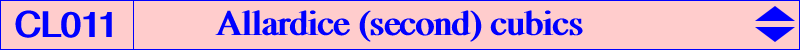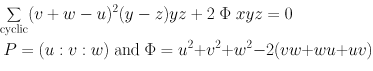The Allardice (second) cubic A2(P) is the anticomplement of the Allardice (first) cubic A1(P). A2(P) is a nK with root G, with pole the barycentric square of the anticomplement of P. It contains the anticomplement aP of P (singular), the points at infinity of the sidelines of ABC. Thus A2(P) is cK(#aP, G). When P lies on the Steiner inscribed ellipse, A2(P) is a nK0+. The general equation of A2(P) is :A2(X2) is K015 Tucker nodal cubic. A2(X115) = K052 is the most remarkable.